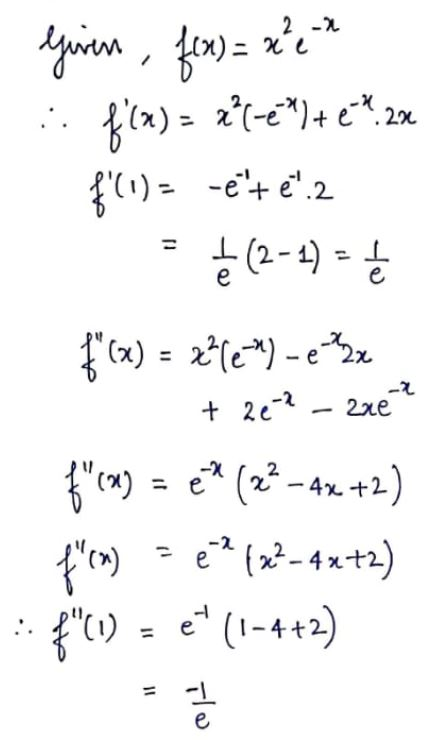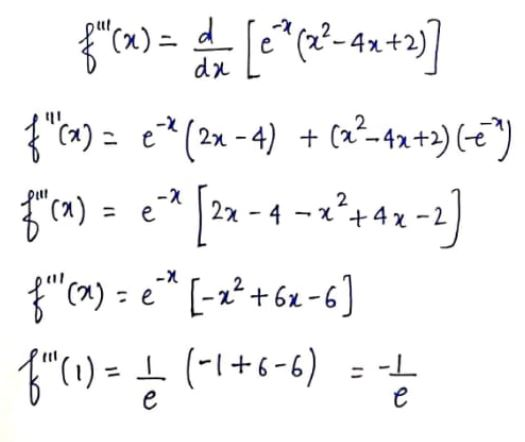# calculate the Taylor polynomials T2 and T3 centered at x = a for the given function and value of a. f (x) = x2e −x , a = 1

Question
8 views

calculate the Taylor polynomials T2 and T3 centered at x = a for the given function and value of a. f (x) = x2e
−x , a = 1

check_circle

Step 1...

### Want to see the full answer?

See Solution

#### Want to see this answer and more?

Solutions are written by subject experts who are available 24/7. Questions are typically answered within 1 hour.*

See Solution
*Response times may vary by subject and question.
Tagged in